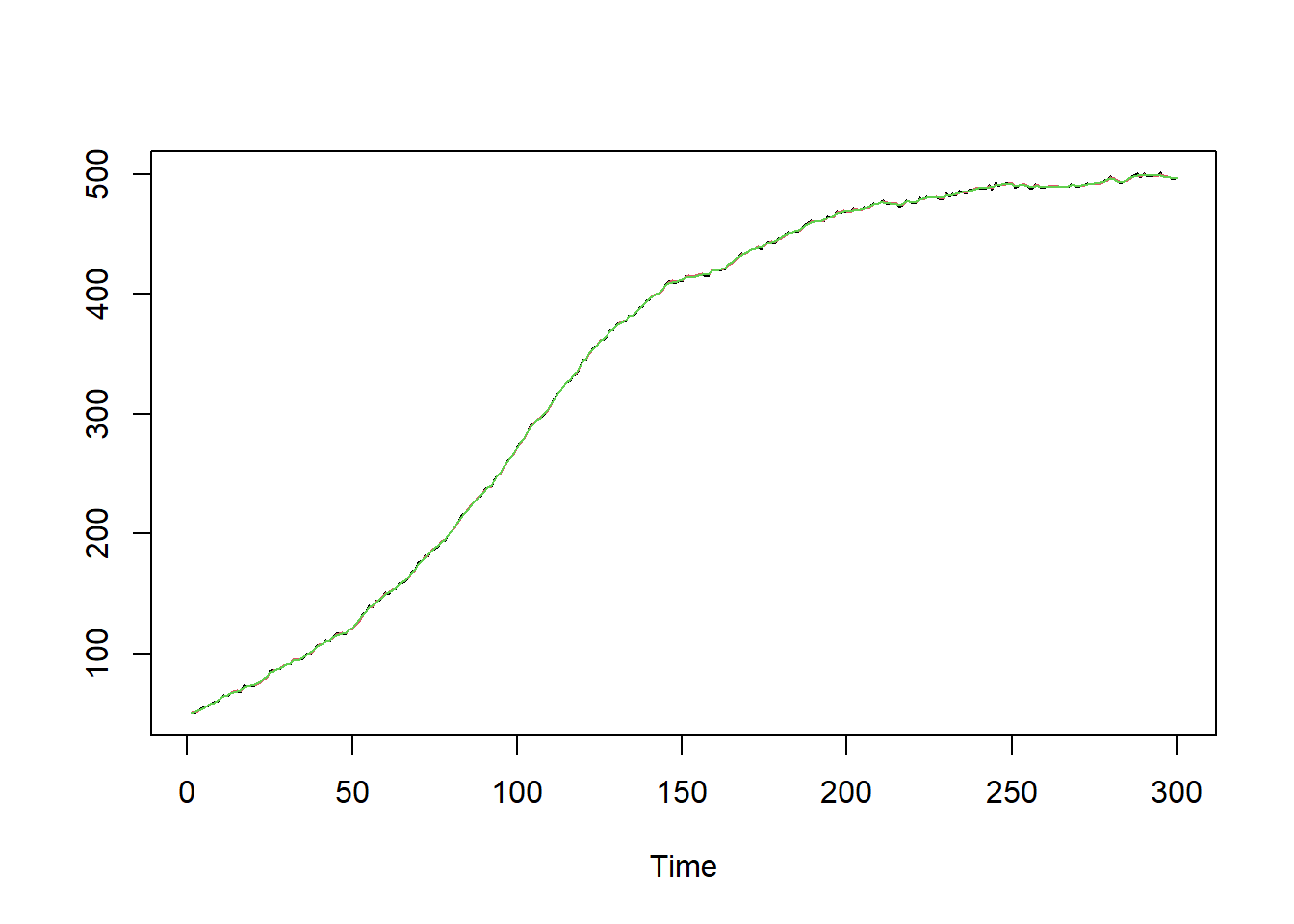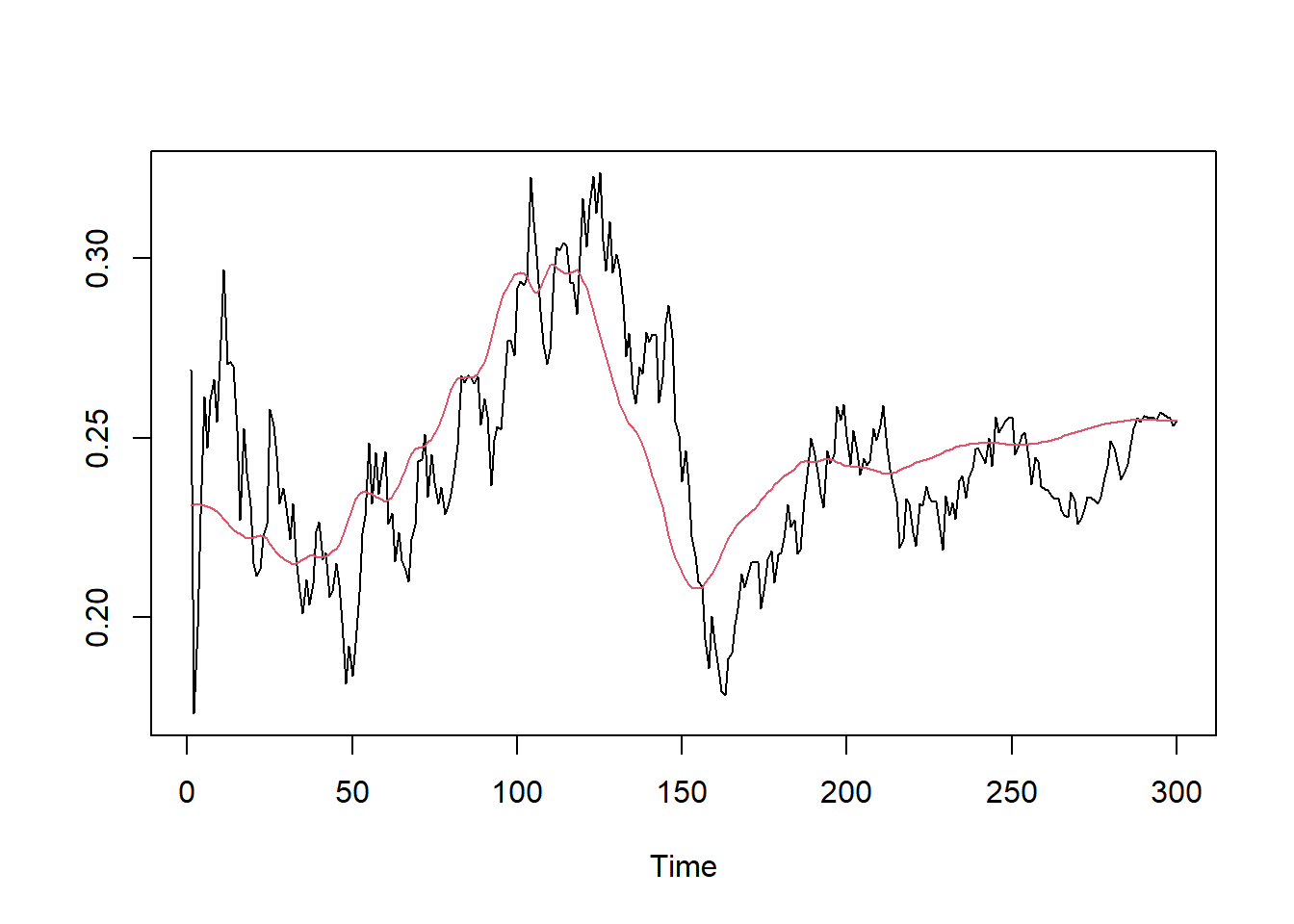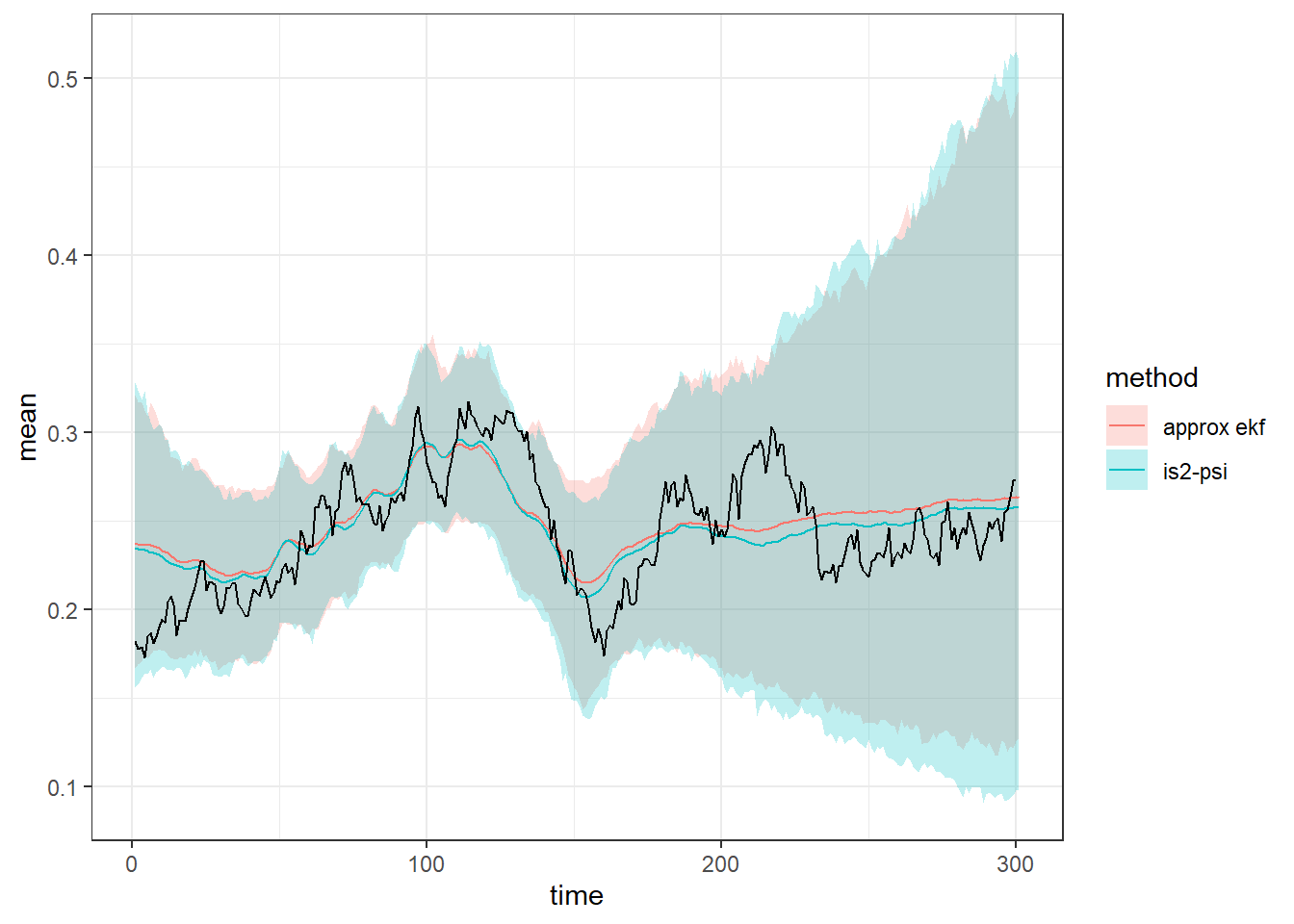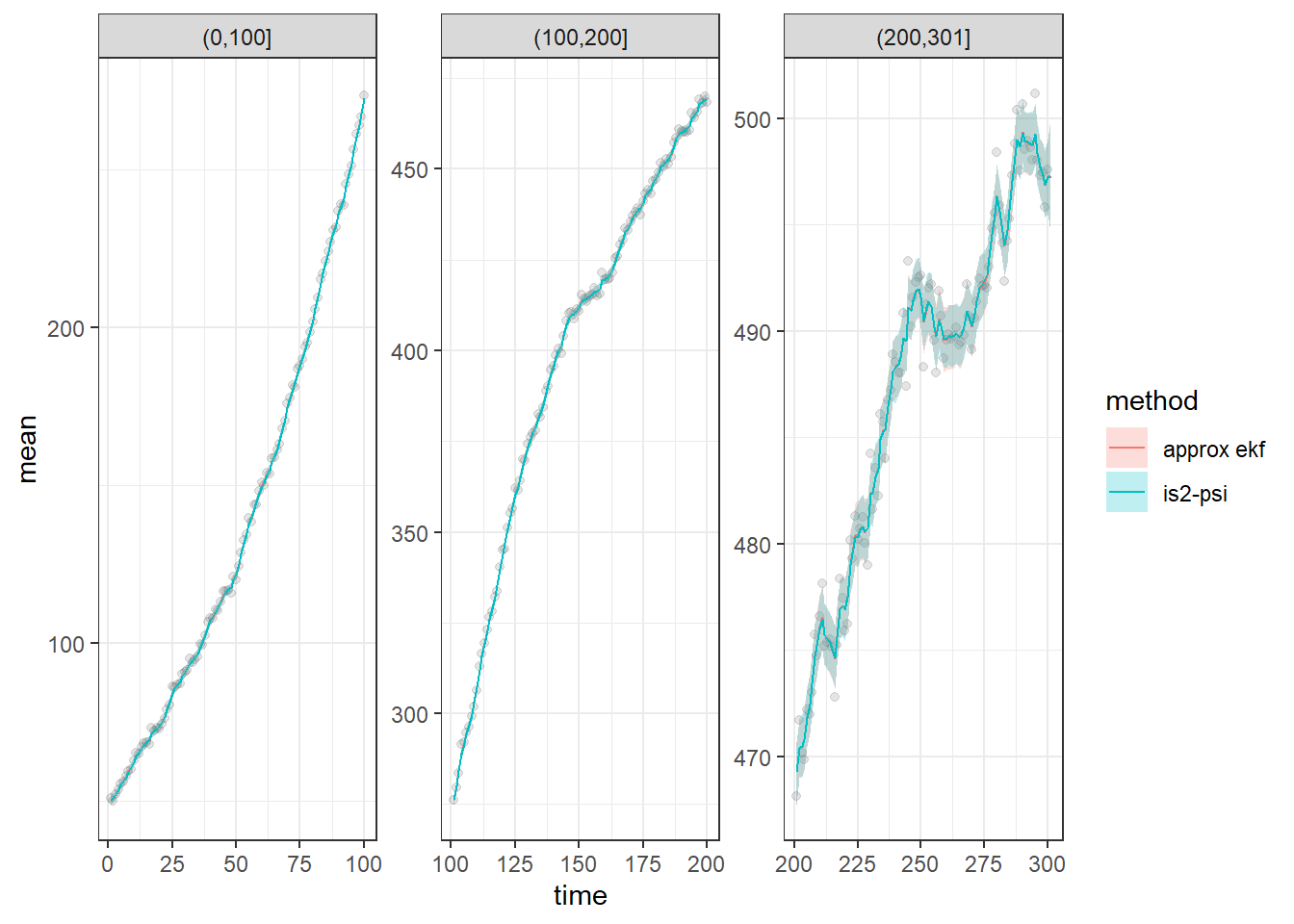## Introduction

This vignette shows how to model general non-linear state space models with bssm. The general non-linear Gaussian model in bssm has following form:

$y_t = Z(t, \alpha_t, \theta) + H(t, \alpha_t, \theta)\epsilon_t,\\ \alpha_{t+1} = T(t, \alpha_t, \theta) + R(t, \alpha_t, \theta)\eta_t,\\ \alpha_1 \sim N(a_1(\theta), P_1(\theta)),$ with $$t=1,\ldots, n$$, $$\epsilon_t \sim N(0,\textrm{I}_p)$$, and $$\eta \sim N(0,\textrm{I}_k)$$. Here vector $$\theta$$ contains the unknown model parameters.

As some of the model matrices may depend on the current state $$\alpha_t$$, constructing for example $$T(t,\alpha_t,\theta)$$ by calling user-defined R function is not feasible, as this should be done repeatedly within the particle filter which would negate the benefits of the whole C++ implementation of the particle filter and Markov chain Monte Carlo. Therefore the functions $$T(\cdot)$$, $$H(\cdot)$$, $$T(\cdot)$$, $$R(\cdot)$$,$$a_1(\cdot)$$, $$P_1(\cdot)$$, as well as functions defining the Jacobians of $$Z(\cdot)$$ and $$T(\cdot)$$ and the prior distribution for $$\theta$$ must be defined by user as a external pointers to C++ functions. For the log-density of theta, we can call R’s own C-level density functions, for example R::dnorm (see the template for an example).

As an example, a logistic growth model of form $y_t = p_t + \epsilon_t,\\ p_{t+1} = K p_t \frac{\exp(r_t dt)}{K + p_t (\exp(r_tdt ) - 1)} + \xi_t,\\ r_t = \frac{\exp{r'_t}}{1 + \exp{r'_t}},\\ r'_{t+1} = r'_t + \eta_t,$ with constant carrying capacity $$K = 500$$, initial population size $$p_1 = 50$$, initial growth rate on logit scale $$r'_1 = -1.5$$, $$dt = 0.1$$, $$\xi \sim N(0,1)$$, $$\eta \sim N(0,0.05)$$, and $$\epsilon \sim N(0, 1)$$.

Let’s first simulate some data, with $$\sigma_r=\sigma_p=0$$:

set.seed(1)

p1 <- 50 # population size at t = 1
K <- 500 # carrying capacity
H <- 1 # standard deviation of obs noise
R_1 <- 0.05 # standard deviation of the noise on logit-growth
R_2 <- 1 # standard deviation of the noise in population level
#sample time
dT <- .1

#observation times
t <- seq(0.1, 30, dT)
n <- length(t)
r <- plogis(cumsum(c(-1.5, rnorm(n - 1, sd = R_1))))
p <- numeric(n)
p <- p1
for(i in 2:n)
p[i] <- rnorm(1, K * p[i-1] * exp(r[i-1] * dT) / (K + p[i-1] * (exp(r[i-1] * dT) - 1)), R_2)
# observations
y <- p + rnorm(n, 0, H)

## Model in bssm

The functions determining the model need to be written in C++. Some example models which can be used as a template are given by the function cpp_example_model which returns pointers usable as an input to nlg_ssm. For this growth model, we could call cpp_example_model("nlg_growth"). In general, you need to define the functions matching the model components, log-density of the prior and few other functions. For example, in case of our model, the function T_fn defines the state transition function $$T(\cdot)$$:

// [[Rcpp::export]]
arma::vec T_fn(const unsigned int t, const arma::vec& alpha, const arma::vec& theta,
const arma::vec& known_params, const arma::mat& known_tv_params) {

double dT = known_params(0);
double K = known_params(1);

arma::vec alpha_new(2);
alpha_new(0) = alpha(0);
double r = exp(alpha(0)) / (1.0 + exp(alpha(0)));
alpha_new(1) = K * alpha(1) * exp(r * dT) /
(K + alpha(1) * (exp(r * dT) - 1));
return alpha_new;
}

The name of this function does not matter, but it should always return Armadillo vector (arma::vec), and have the same signature (i.e. the order and types of the function’s parameters) should always be like above, even though some of the parameters were not used in the body of the function. Note that all of these functions can also depend on some known parameters, given as known_params (vector) and known_tv_params (matrix) arguments to ssm_nlg function (which are then passed to individual C++ snippets). For details of using Armadillo, see Armadillo documentation. After defining the appropriate model functions, the cpp file should also contain a function for creating external pointers for the aforementioned functions. Why this is needed is more technical issue, but fortunately you can just copy the function from the example file without any modifications.

After creating the file for C++ functions, you need to compile the file using Rcpp1:

Rcpp::sourceCpp("ssm_nlg_template.cpp")
## Warning in normalizePath(path.expand(path), winslash, mustWork):
## path="C:/Users/jvhels/AppData/Local/Temp/RtmpOcbFRh/Rbuild40c068197473/bssm/vignettes/../inst/include":
## The system cannot find the file specified
pntrs <- create_xptrs()

This takes a few seconds. let’s define our initial guess for $$\theta$$, the logarithms of the standard deviations of observational and process level noise, and define the prior distribution for $$\alpha_1$$ (we use log-scale in sampling for efficiency reasons, but define priors for the standard deviations, see the template file at the appendix):

initial_theta <- c(log_H = 0, log_R1 = log(0.05), log_R2 = 0)

# dT, K, a1 and the prior variances of 1st and 2nd state (logit r and and p)
known_params <- c(dT = dT, K = K, a11 = -1, a12 = 50, P11 = 1, P12 = 100)

If you have used line // [[Rcpp::export]] before the model functions, you can now test that the functions work as intended:

T_fn(0, c(100, 200), initial_theta, known_params, matrix(1))
##         [,1]
## [1,] 100.000
## [2,] 212.111

Now the actual model object using ssm_nlg:

library("bssm")
model <- ssm_nlg(y = y, a1=pntrs$a1, P1 = pntrs$P1,
Z = pntrs$Z_fn, H = pntrs$H_fn, T = pntrs$T_fn, R = pntrs$R_fn,
Z_gn = pntrs$Z_gn, T_gn = pntrs$T_gn,
theta = initial_theta, log_prior_pdf = pntrs$log_prior_pdf, known_params = known_params, known_tv_params = matrix(1), n_states = 2, n_etas = 2, state_names = c("logit_r", "p")) Let’s first run Extended Kalman filter and smoother using our initial guess for $$\theta$$: out_filter <- ekf(model) out_smoother <- ekf_smoother(model) ts.plot(cbind(y, out_filter$att[, 2],
out_smoother$alphahat[, 2]), col = 1:3)ts.plot(plogis(cbind(out_filter$att[, 1],
out_smoother$alphahat[, 1])), col = 1:2)## Markov chain Monte Carlo For parameter inference, we can perform full Bayesian inference with . There are multiple choices for the MCMC algorithm in the package, and here we will use the default choice, which is an approximate MCMC with $$\psi$$-APF based importance sampling correction . Let us compare this approach with EKF-based approximate MCMC (the former is unbiased, whereas latter is not). Due to package check requirements in CRAN, we use only small number of iterations: # Cholesky of initial proposal matrix (taken from previous runs) # used here to speed up convergence due to the small number of iterations S <- matrix(c(0.13, 0.13, -0.11, 0, 0.82, -0.04, 0, 0, 0.16), 3, 3) mcmc_is <- run_mcmc(model, iter = 6000, burnin = 1000, particles = 10, mcmc_type = "is2", sampling_method = "psi", S = S) mcmc_ekf <- run_mcmc(model, iter = 6000, burnin = 1000, mcmc_type = "ekf", S = S) summary(mcmc_is, return_se = TRUE) ## variable Mean SE SD 2.5% 97.5% ESS ## 1 log_H 0.14949688 0.003365320 0.07607332 -0.01165514 0.2926221 511 ## 2 log_R1 -3.03473610 0.024811411 0.53050836 -4.18811749 -2.1128308 457 ## 3 log_R2 -0.01087708 0.005457274 0.11887128 -0.26668791 0.2174341 474 ## SE_IS ESS_IS ## 1 0.001086538 3945 ## 2 0.007734927 4834 ## 3 0.001691797 2926 summary(mcmc_ekf, return_se = TRUE) ## variable Mean SE SD 2.5% 97.5% ESS ## 1 log_H 0.13888612 0.003718327 0.07969306 -0.03525923 0.2846795 459 ## 2 log_R1 -3.20977925 0.047232416 0.66871031 -5.11104168 -2.2613285 200 ## 3 log_R2 0.01288363 0.005615988 0.11664585 -0.21490113 0.2297731 431 Using the as.data.frame method we can convert the state samples to a data frame for further processing with the dplyr package (we could do this automatically with summary method as well): library("dplyr") library("diagis") d1 <- as.data.frame(mcmc_is, variable = "states") d2 <- as.data.frame(mcmc_ekf, variable = "states") d1$method <- "is2-psi"
d2$method <- "approx ekf" r_summary <- rbind(d1, d2) |> filter(variable == "logit_r") |> group_by(time, method) |> summarise( mean = weighted_mean(plogis(value), weight), lwr = weighted_quantile(plogis(value), weight, 0.025), upr = weighted_quantile(plogis(value), weight, 0.975)) ## summarise() has grouped output by 'time'. You can override using the ## .groups argument. p_summary <- rbind(d1, d2) |> filter(variable == "p") |> group_by(time, method) |> summarise( mean = weighted_mean(value, weight), lwr = weighted_quantile(value, weight, 0.025), upr = weighted_quantile(value, weight, 0.975)) ## summarise() has grouped output by 'time'. You can override using the ## .groups argument. Above we used the weighted versions of mean and quantile functions provided by the diagis package as our IS-MCMC algorithm produces weighted samples of the posterior. Alternatively, we could have used argument output_type = "summary", in which case the run_mcmc returns posterior means and covariances of the states instead of samples (these are computed using the full output of particle filter so these estimates are more accurate). Using ggplot2 we can compare our two estimation methods: library("ggplot2") ggplot(r_summary, aes(x = time, y = mean)) + geom_ribbon(aes(ymin = lwr, ymax = upr, fill = method), colour = NA, alpha = 0.25) + geom_line(aes(colour = method)) + geom_line(data = data.frame(mean = r, time = seq_along(r))) + theme_bw()p_summary$cut <- cut(p_summary$time, c(0, 100, 200, 301)) ggplot(p_summary, aes(x = time, y = mean)) + geom_point(data = data.frame( mean = y, time = seq_along(y), cut = cut(seq_along(y), c(0, 100, 200, 301))), alpha = 0.1) + geom_ribbon(aes(ymin = lwr, ymax = upr, fill = method), colour = NA, alpha = 0.25) + geom_line(aes(colour = method)) + theme_bw() + facet_wrap(~ cut, scales = "free")In this example, EKF approximation performs well compared to exact method, while being considerably faster: mcmc_is$time
##    user  system elapsed
##   42.15    0.00   42.24
mcmc_ekf\$time
##    user  system elapsed
##    5.69    0.00    5.70

## Appendix

This is the full ssm_nlg_template.cpp file (identical with nlg_growth accessible with cpp_example_model):

// A template for building a general non-linear Gaussian state space model
// Here we define an univariate growth model (see vignette growth_model)

// [[Rcpp::interfaces(r, cpp)]]

// Unknown parameters theta:
// theta(0) = log(H)
// theta(1) = log(R_1)
// theta(2) = log(R_2)

// Function for the prior mean of alpha_1
// [[Rcpp::export]]
arma::vec a1_fn(const arma::vec& theta, const arma::vec& known_params) {

arma::vec a1(2);
a1(0) = known_params(2);
a1(1) = known_params(3);
return a1;
}
// Function for the prior covariance matrix of alpha_1
// [[Rcpp::export]]
arma::mat P1_fn(const arma::vec& theta, const arma::vec& known_params) {

arma::mat P1(2, 2, arma::fill::zeros);
P1(0,0) = known_params(4);
P1(1,1) = known_params(5);
return P1;
}

// Function for the observational level standard deviation
// [[Rcpp::export]]
arma::mat H_fn(const unsigned int t, const arma::vec& alpha, const arma::vec& theta,
const arma::vec& known_params, const arma::mat& known_tv_params) {
arma::mat H(1,1);
H(0, 0) = exp(theta(0));
return H;
}

// Function for the Cholesky of state level covariance
// [[Rcpp::export]]
arma::mat R_fn(const unsigned int t, const arma::vec& alpha, const arma::vec& theta,
const arma::vec& known_params, const arma::mat& known_tv_params) {
arma::mat R(2, 2, arma::fill::zeros);
R(0, 0) = exp(theta(1));
R(1, 1) = exp(theta(2));
return R;
}

// Z function
// [[Rcpp::export]]
arma::vec Z_fn(const unsigned int t, const arma::vec& alpha, const arma::vec& theta,
const arma::vec& known_params, const arma::mat& known_tv_params) {
arma::vec tmp(1);
tmp(0) = alpha(1);
return tmp;
}
// Jacobian of Z function
// [[Rcpp::export]]
arma::mat Z_gn(const unsigned int t, const arma::vec& alpha, const arma::vec& theta,
const arma::vec& known_params, const arma::mat& known_tv_params) {
arma::mat Z_gn(1, 2);
Z_gn(0, 0) = 0.0;
Z_gn(0, 1) = 1.0;
return Z_gn;
}

// T function
// [[Rcpp::export]]
arma::vec T_fn(const unsigned int t, const arma::vec& alpha, const arma::vec& theta,
const arma::vec& known_params, const arma::mat& known_tv_params) {

double dT = known_params(0);
double K = known_params(1);

arma::vec alpha_new(2);
alpha_new(0) = alpha(0);
double r = exp(alpha(0)) / (1.0 + exp(alpha(0)));
alpha_new(1) = K * alpha(1) * exp(r * dT) /
(K + alpha(1) * (exp(r * dT) - 1));
return alpha_new;
}

// Jacobian of T function
// [[Rcpp::export]]
arma::mat T_gn(const unsigned int t, const arma::vec& alpha, const arma::vec& theta,
const arma::vec& known_params, const arma::mat& known_tv_params) {

double dT = known_params(0);
double K = known_params(1);

double r = exp(alpha(0)) / (1 + exp(alpha(0)));

double tmp = exp(r * dT) / std::pow(K + alpha(1) * (exp(r * dT) - 1), 2);

arma::mat Tg(2, 2);
Tg(0, 0) = 1.0;
Tg(0, 1) = 0;
Tg(1, 0) = dT * K * alpha(1) * (K - alpha(1)) * tmp * r / (1 + exp(alpha(0)));
Tg(1, 1) = K * K * tmp;

return Tg;
}

// log-prior pdf for theta
// [[Rcpp::export]]
double log_prior_pdf(const arma::vec& theta) {

// weakly informative half-N(0, 4) priors.

// Note that the sampling is on log-scale,
// so we need to add jacobians of the corresponding transformations
// we could also sample on natural scale with check such as
// if(arma::any(theta < 0)) return -std::numeric_limits<double>::infinity();
// but this would be less efficient.

// You can use R::dnorm and similar functions, see, e.g.
// https://teuder.github.io/rcpp4everyone_en/220_dpqr_functions.html
double log_pdf =
R::dnorm(exp(theta(0)), 0, 2, 1) +
R::dnorm(exp(theta(1)), 0, 2, 1) +
R::dnorm(exp(theta(2)), 0, 2, 1) +
arma::accu(theta); //jacobian term

return log_pdf;
}

// Create pointers, no need to touch this if
// you don't alter the function names above
// [[Rcpp::export]]
Rcpp::List create_xptrs() {

// typedef for a pointer of nonlinear function of model equation returning vec (T, Z)
typedef arma::vec (*nvec_fnPtr)(const unsigned int t, const arma::vec& alpha,
const arma::vec& theta, const arma::vec& known_params, const arma::mat& known_tv_params);
// typedef for a pointer of nonlinear function returning mat (Tg, Zg, H, R)
typedef arma::mat (*nmat_fnPtr)(const unsigned int t, const arma::vec& alpha,
const arma::vec& theta, const arma::vec& known_params, const arma::mat& known_tv_params);

// typedef for a pointer returning a1
typedef arma::vec (*a1_fnPtr)(const arma::vec& theta, const arma::vec& known_params);
// typedef for a pointer returning P1
typedef arma::mat (*P1_fnPtr)(const arma::vec& theta, const arma::vec& known_params);
// typedef for a pointer of log-prior function
typedef double (*prior_fnPtr)(const arma::vec& theta);

return Rcpp::List::create(
Rcpp::Named("a1_fn") = Rcpp::XPtr<a1_fnPtr>(new a1_fnPtr(&a1_fn)),
Rcpp::Named("P1_fn") = Rcpp::XPtr<P1_fnPtr>(new P1_fnPtr(&P1_fn)),
Rcpp::Named("Z_fn") = Rcpp::XPtr<nvec_fnPtr>(new nvec_fnPtr(&Z_fn)),
Rcpp::Named("H_fn") = Rcpp::XPtr<nmat_fnPtr>(new nmat_fnPtr(&H_fn)),
Rcpp::Named("T_fn") = Rcpp::XPtr<nvec_fnPtr>(new nvec_fnPtr(&T_fn)),
Rcpp::Named("R_fn") = Rcpp::XPtr<nmat_fnPtr>(new nmat_fnPtr(&R_fn)),
Rcpp::Named("Z_gn") = Rcpp::XPtr<nmat_fnPtr>(new nmat_fnPtr(&Z_gn)),
Rcpp::Named("T_gn") = Rcpp::XPtr<nmat_fnPtr>(new nmat_fnPtr(&T_gn)),
Rcpp::Named("log_prior_pdf") =
Rcpp::XPtr<prior_fnPtr>(new prior_fnPtr(&log_prior_pdf)));

}
Helske, Jouni. n.d. diagis: Diagnostic Plot and Multivariate Summary Statistics of Weighted Samples from Importance Sampling. https://github.com/helske/diagis.
Vihola, Matti, Jouni Helske, and Jordan Franks. 2020. “Importance Sampling Type Estimators Based on Approximate Marginal MCMC.” Scandinavian Journal of Statistics. https://doi.org/10.1111/sjos.12492.
Wickham, Hadley. 2016. Ggplot2: Elegant Graphics for Data Analysis. Springer-Verlag New York. https://ggplot2.tidyverse.org.
Wickham, Hadley, Romain François, Lionel Henry, and Kirill Müller. 2020. Dplyr: A Grammar of Data Manipulation.

1. As repeated calls to compile same cpp file can sometimes lead to memory issues, it is good practice to define unique cache directory using the cacheDir` argument(see issue in Github). But the CRAN does not like this approach so we do not use it here.↩︎